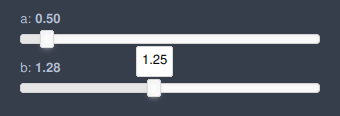# How to link two sliders together

I’m trying to link two sliders together in such a way that their values won’t overlap. Here, I used a callback function to modify the parameters on the second slider.

``````import panel as pn
a = pn.widgets.FloatSlider(name="a", start=0.4, end=1.6, value=0.5)
b = pn.widgets.FloatSlider(name="b", start=1, end=2, value=1.5)
def callback(*events):
for event in events:
if event.name == 'value':
_min = 1.3 * event.new
_max = 4 * event.new
b.start = _min
b.end = _max
if b.val < _min:
b.val = _min
elif b.val > _max:
b.val = _max

a.param.watch(callback, ["start", 'value'], onlychanged=False)
pn.Column(a, b)
``````

I’ve almost did it, however the label of the second does not update, hence the user might get misleading information. What can I do?I am not sure if .val is a valid acronym for .value.

Maybe try
b.value?

You are right about `value`. However, the label still doesn’t updateIt does update when you reach the threshold; the reason why you’re seeing the slider move around is because you update the .start + .end, but the .value doesn’t get triggered unless it passes the logic.
e.g. if you remove the logic

``````import panel as pn
pn.extension()
a = pn.widgets.FloatSlider(name="a", start=0.4, end=1.6, value=0.5)
b = pn.widgets.FloatSlider(name="b", start=1, end=2, value=1.5)

def callback(*events):
for event in events:
if event.name == 'value':
_min = 1.3 * event.new
_max = 4 * event.new
b.start = _min
b.end = _max
b.value = _max

a.param.watch(callback, ["start", 'value'])
pn.Column(a, b)
``````